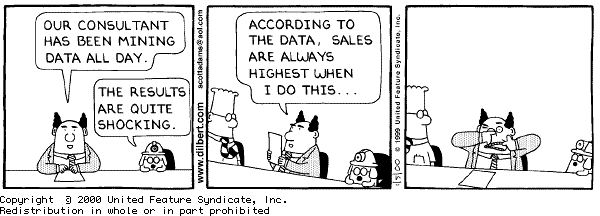# CSCE 782: Problem Set 7

## Due: 14 November 2000

Problem 1 (10%): Draw the game matrix for a 2-person game, each person having three choices: A, B, C, where (A,A) is a Nash equilibrium, and (C,C) is the pareto-optimal choice.

Problem 2 (50%): Find the dominant strategy (if any), pareto optima (if any), and Nash equilibrium (use mixed strategies if needed) for each of the following game matrices:

1.  C D A 1,2 3,4 B 5,6 7,8

2.  C D A 1,1 1,2 B 1,2 2,2

3.  C D A 1,-1 3,0 B 4,2 0,1

4.  C D A 7,4 3,3 B 0,0 4,7

5.  C D A 4,4 3,3 B 0,0 4,7

Problem 3 (15%):Two players, A and B, are playing a game where each one of them has 5 dollar bills and decides how many to throw on the floor. Both players make their throws simultaneously. If total number of dollars on the floor is even, then A gets all the money. If it is odd, then B gets all the money. Draw the game matrix that corresponds to this game and determine the selfish strategy of these agents. Explain your reasoning. How would your solution change if the player's had a logarithmic utility of money? That is, if their utility for x dollars was log(x), instead of x.

Problem 4 (10%): Why is the Borda count a preferred voting mechanism? That is, what properties does it obey that make it a fairer solution?

Problem 5 (15%): Give an example situation where a strategic voter in a plurality vote should (being smart) decide not to vote its true preference. Specifically, show the set of agents, the set of outcomes, and the agents' preferences over the outcomes (you can give these in table form using preferences, as we did in class for the mathematicians). Also, explain why the agent should make that chose.Jose M. Vidal